Subsections

# 2.3 Horizontal stresses

## 2.3.1 Background

Vertical (effective) stress is not enough to define the state of stress in a solid. Stresses in horizontal direction are very often different to the stress in vertical direction. The state of stress can be fully defined by the “principal stresses”. These are three independent normal stresses in directions all perpendicular to each other. A stress is a principal stress if there is no shear stress on the plane in which it is applied. Total vertical stressmay not be a principal stress, although in most cases it is. If vertical stress is a principal stress, then the two other principal stresses are horizontal. The maximum principal stress in the horizontal case isand the minimum horizontal stress is, such that(Figure 2.20).We have seen that total vertical stress is mostly a function of overburden and depth. Now, what determines horizontal stresses? There is not an easy answer for that. Many variables affect and limit horizontal stresses. First, there are “background” horizontal stresses that develop due to the weight of overburden, its compaction, and “pushing sideways” effect. For example, water pushes sideways with all its weight (pressure is the same in all directions). Solids push sideways with a fraction of their weight. Second, horizontal stresses may deviate from background stresses - to be either more or less compressive. Tectonic plate movements are the main contributors to variations of horizontal stress. Convergent plates increase horizontal compression. Divergent plates decrease horizontal compression. Shear stresses develop at transform boundaries. Other factors include topography, crustal thickening/thinning, mass density anomalies, buoyancy forces, and lithospheric flexure (similar effect of a loaded slab).The direction of maximum horizontal stress varies with location around the Earth's crust. Tectonic plate movements are the main contributors the affect the direction of maximum horizontal stress (See Figure 2.22).## 2.3.2 Geological indicators

Geological formations can be indicators of “paleo-stress” direction, that is, the stress that caused such feature at a particular time. The paleo-stress, however, may be different from the current state of stress in magnitude and direction. Keep in mind that the Earth crust today is the results of millions of years of plate movement, smashing, and thinning/thickening (see this great animation of the Earth crust evolution since the Precambrian https://dinosaurpictures.org/ancient-earth#150.) The orientation of fault planes is an indicator of the state of stress that caused such fault. This topic will be seen later in “Fault stability" analysis. Folding direction also can give an idea of the horizontal stress that produced such fold. Natural fractures can be indicators of shear or open mode fractures. Both are related to the orientation of the state of stress. Earthquake focal mechanisms tell about the polarity of waves emitted by rock failure, where rock failure is also related to the orientation of the state of stress (to be covered later).

Magmatic and sedimentary dikes are natural hydraulic fractures. They form when a pressurized fluid/sediment mixture opens the subsurface and props the recently opened space with crystallized magma or sediments. Dikes, like any other hydraulic fracture, open up preferentially against the least principal stress.## 2.3.3 In-situ measurement

The only way to know the current magnitude (and direction) of the minimum horizontal stress(if) is to measure it. Subsurface measurements ofare based in hydraulic fracturing methods (to be seen in detail later in the course, Chapter 7). Three types of hydraulic fracture tests are:

• Extended leak-off test: performed during drilling with drilling mud. The minimum principal stressis measured at about the value of the fracture closure pressure.
• Mini-frac test: Performed for completion purposes before the main hydraulic fracture treatment with fracturing fluid. The analysis to obtainis based on the same principles of the extended leak-off test.
• Step-rate test: Used for continuous fluid injection. The formation parting pressure (when a hydraulic fracture forms) is related but higher than.

## 2.3.4 Stress Regimes

The variations of horizontal stress in the lithosphere give rise to three types of stress regimes, depending on the relative magnitude of horizontal stress with respect to vertical stress (Table 2.1). Stress is a tensor. Every stress tensor has three characteristic values called principal stresses,, and(eigenvalues https://en.wikipedia.org/wiki/Eigenvalues_and_eigenvectors ). The principal stressis the maximum normal stress value in a given direction (maximum total principal stress). The principal stressis the minimum normal stress value in a given direction (minimum total principal stress). The principal stressis an intermediate stress value at a direction perpendicular toand. Figure 2.24 shows a map of the United States (lower 48 states) with the three types of stress regimes explained in Table 2.1. Figure 2.25 shows the variation of stress regimes and horizontal stress diection in Texas.

 Stress Regime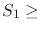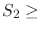Normal FaultingStrike-Slip FaultingReverse/Thrust Faulting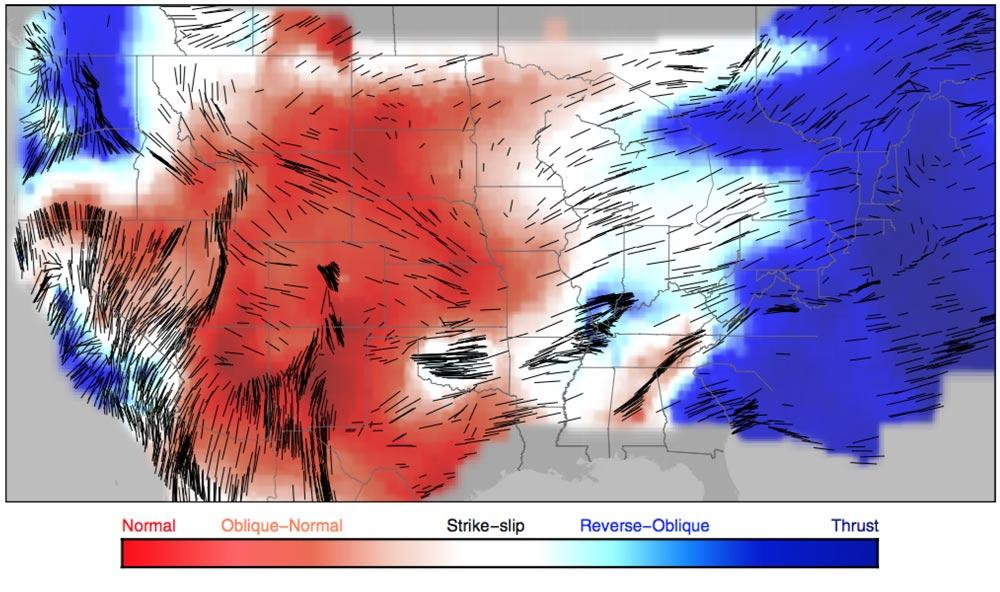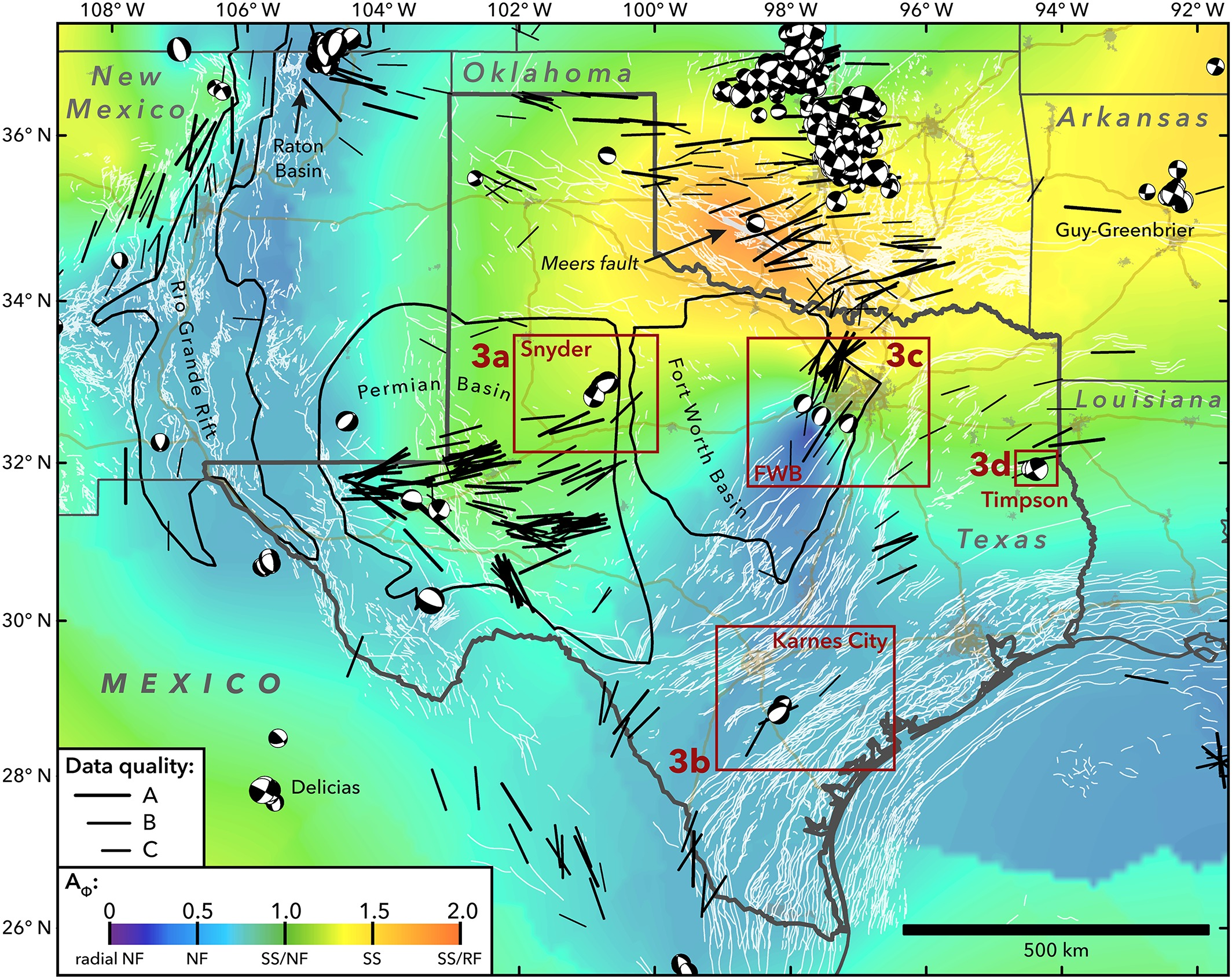### 2.3.4.1 Normal faulting stress regime

Normal faulting occurs in tectonically passive or extensional environments, such that, where,,. The minimum horizontal stresscan be as low as% of, but not much lower. If lower, sedimentary strata fail creating normal faults due to large differences between effective stressesand. Hydraulic fractures in this environment would be vertical (perpendicular todirection). Most hydrocarbon-producing basins in the USA are in normal faulting stress regimes.### 2.3.4.2 Strike slip faulting stress regime

Strike slip faulting occurs in “mild” tectonically compressive environments, such thatwhere,, and. These stress regimes cause strike-slip faults whensurpassesabove the frictional limit. Hydraulic fractures in this environment are vertical and perpendicular todirection. Some giant oil fields in the middle East are in strike-slip stress regime.

### 2.3.4.3 Reverse faulting stress regime

Reverse faulting occurs in “strong” tectonically compressive environments, such that, where,,. These stress regimes cause reverse and thrust faults whensurpassesabove the failure limit. Hydraulic fractures in this environment are horizontal! (perpendicular todirection). Some unconventional fields in Argentina and Australia are in reverse faulting stress-regime at specific depths.

## 2.3.5 Ideal orientation of open-mode fractures

Why is the shape of an inflated balloon spherical (Figure 2.27)? Even if you were to inflate the ballon under water, it would be still spherical. The reason is that pressure around the balloon is the same in all directions. It would be the atmospheric pressure in air at surface conditions or the water pressureat seafloor conditions. Now, what would be the shape of a balloon inflated under several layers of sediments? Would it be spherical or not?

The previous section anticipated the hydraulic fractures are perpendicular to the direction of the least principal stress. The reason for such behavior responds to the tendency of nature to go through the path of “least amount of energy”. There is no reason to push away rock in a direction of higher stress if there is another direction in which stress is lower and it is easier to push. Thus, hydraulic fractures would be perpendicular to the least principal stress as long asis different and lower thanand. As a result, the shape of a balloon inflated under several layers of sediment would be a flat ellipsoid with smallest axis in the direction of.Let us consider a place in the subsurface like the one shown in Figure 2.27. There are three possible cases for the orientation of a hydraulic fracture depending on the values and orientations of the principal stresses. In Case 1 the least principal stress is horizontal in the direction of axis 2, hence(the subindices of stresses are explained in detail in the next chapter). Case 1 is the one drawn on the top right schematic. In Case 2 the least principal stress is horizontal in the direction of axis 1,. In Case 3 the least principal stress is vertical in the direction of axis 3,.

Given a state of stress, multistage hydraulic fracturing may result or not in independent fracture planes. Consider the example in Figure 2.28 where. The hydraulic fracture planes would always tend to open up againstregardless of the wellbore orientation. Multiple hydraulic fractures would tend to link on a single plane if started from a vertical wellbore or a horizontal wellbore drilled in the direction of. Hydraulic fractures would have much less tendency to link up if started from a horizontal wellbore drilled in the direction of.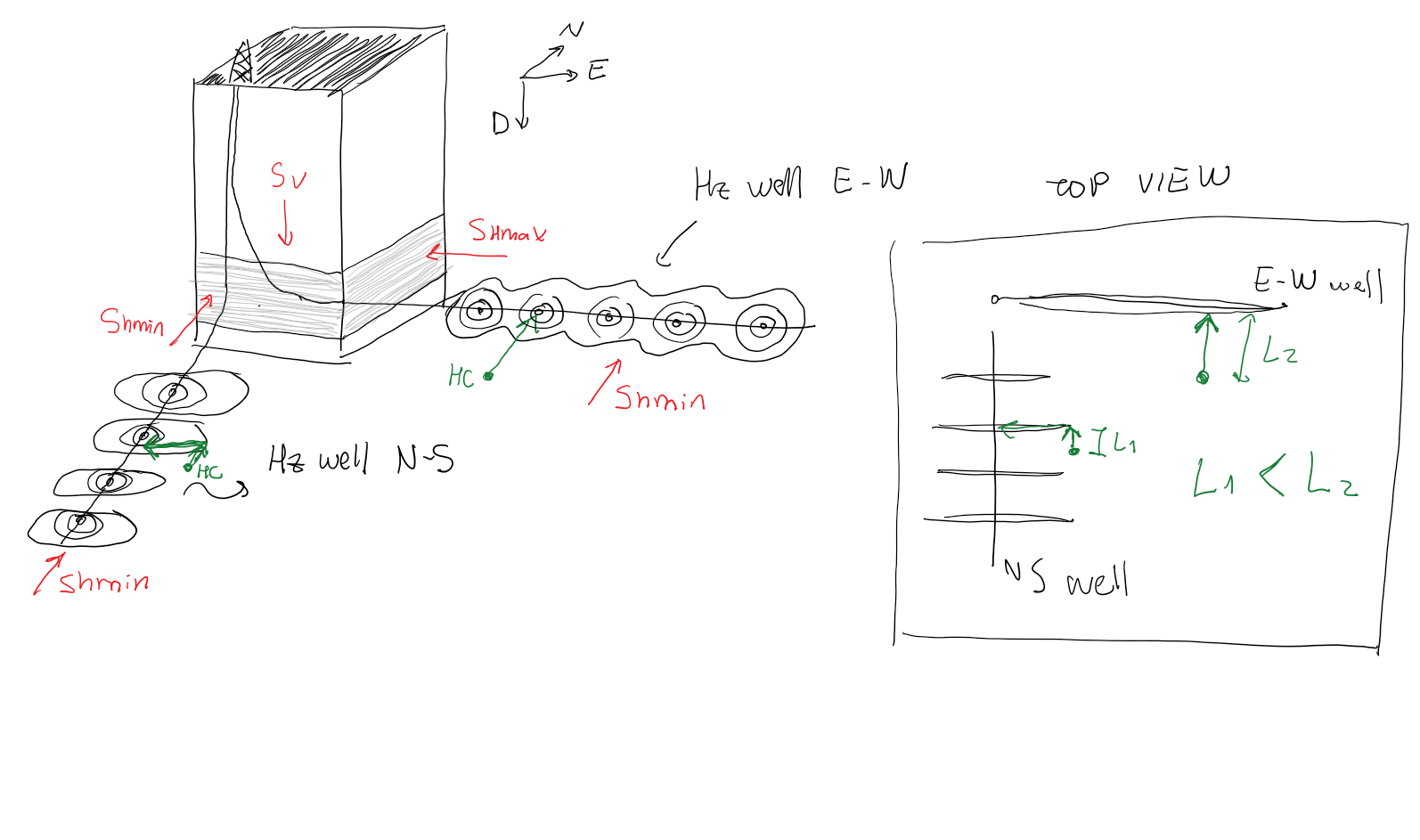For example, the Barnett shale Formation near Dallas-Forth Worth is subjected to a normal faulting stress regime. Furthermore, the maximum horizontal total stressis in NE-SW direction (about 60from the North clockwise - Figure 2.29). As a result, the (least) minimum horizontal stress is oriented 30from the North counter-clockwise. Consequently, hydraulic fractures are expected to be vertical and the preferential orientation of horizontal wellbores for multistage hydraulic fracturing is either 30from the North counter-clockwise in direction NW or 120from the North clockwise in direction SE.